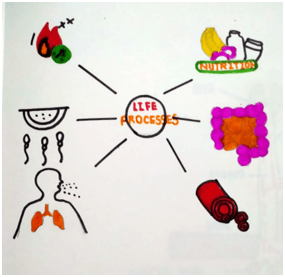# CBSE Diagrams for Class 10 Biology Important for Exams

## CBSE Diagrams for Class 10 Biology

In class 10, biology there are 6 chapters are given in syllabus. Therefore we have added important diagrams from the syllabus. There are 20 important diagrams are added in syllabus. Let’s discuss in brief about the diagrams.

Chapter 6 : Life Processes-

This chapter is considered as one of the large chapter included in the syllabus. There are various important concepts have been given in this chapter. So diagrams are also important. In this chapter we have assimilated total 8 diagrams. In which first diagram included is Life processes, by which students can understand what are the processes are carried out during the life.

After this next diagram is for the reaction of photosynthesis, due to this diagram students can observe how plants prepare their own food.

In the same chapter we can understand the single celled organism amoeba nutrition, therefore amoeba nutrition diagram is included.

The next diagram of the chapter is Respiration and photosynthesis reaction in plant by which students can come to know the two different processes of plants.

Along with these concepts, how the food is transported in plants to different parts of the plants, so we have drawn the diagram of Transport in plants, so students can know about the transporting mechanism in plants.

Next concept is included about the human systems, in which we have drawn the diagram of Components of blood, it helps the student to learn the concept of blood. Afterwards Circulation of blood and Excretory system diagrams have been drawn to study the mechanism of blood circulation and excretion in human body.Figure 1: Life processesFigure 2: Reaction of photosynthesisFigure 3: Amoeba nutritionFigure 4: . Respiration and photosynthesis reaction in plantFigure 5: Transport in plantsFigure 6: Components of bloodFigure 7: Circulation of bloodFigure 8: Excretory system

Chapter 7: Control and Co-ordination

This chapter is mainly divided into 2 parts such as plant hormones and human nervous system. Therefore first we included the diagram of hormones and their functions in plants, so students can observe diagrammatically all the hormones working in plants. When we discuss about the plant hormones then their movement is also important so we have drawn the diagrams of their movement such as Tropic movement diagram and Nastic movement diagram by which how the hormones move in the plant body that can be understood.

The next part of the chapter is about the nervous system for which we have included the diagram of neuron and parts of nervous system such as Nervous system diagram and Brain and coordinating system with this is Endocrine system. Therefore endocrine system diagram is also drawn.Figure 9: Tropic movement diagramFigure 10: Nastic movement diagramFigure 11: Structure of neuronFigure 12: Nervous systemFigure 13: BrainFigure 14: Hormones and their functionsFigure 15: Endocrine system

Chapter 8: How do organisms reproduce?

In this chapter the reproduction of living organisms is shown. In which we have added the important diagram of Regeneration of planaria and Fertilization in plants. These diagrams are ask in exam for 5 marks.Figure 16: Regeneration of planariaFigure 17:Fertilization in plants

Chapter 9: Heredity and evolution

The chapter mainly concerns with how the characters passes from one generation to the next generation, to understand this the scientist Mendel gave Monohybrid cross and Dihybrid cross. Therefore we’ve drawn both the diagrams. Last part of the chapter comes to Blood group detection. Therefore blood group detection diagram is included.Figure 18: Monohybrid cross and Dihybrid crossFigure 19: Blood group detection

Updated: December 6, 2021 — 6:28 pm